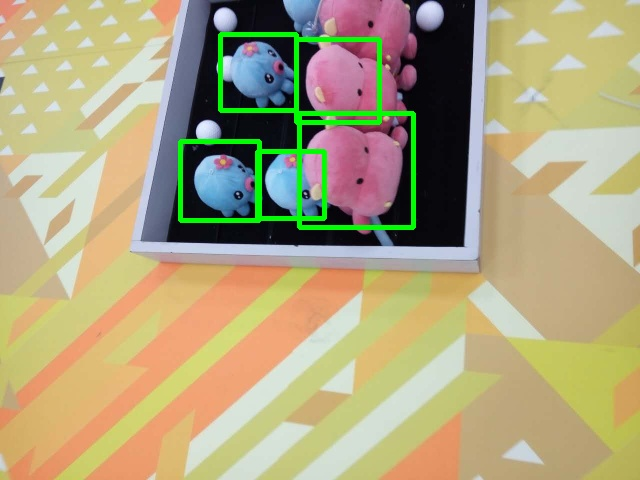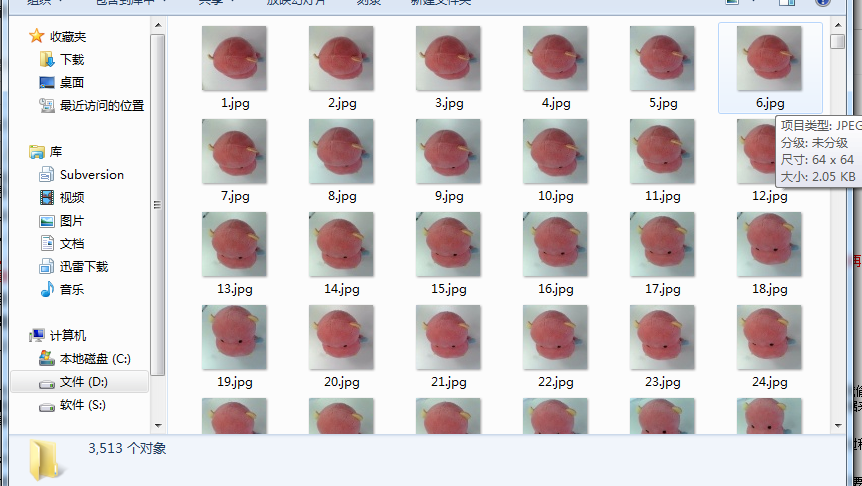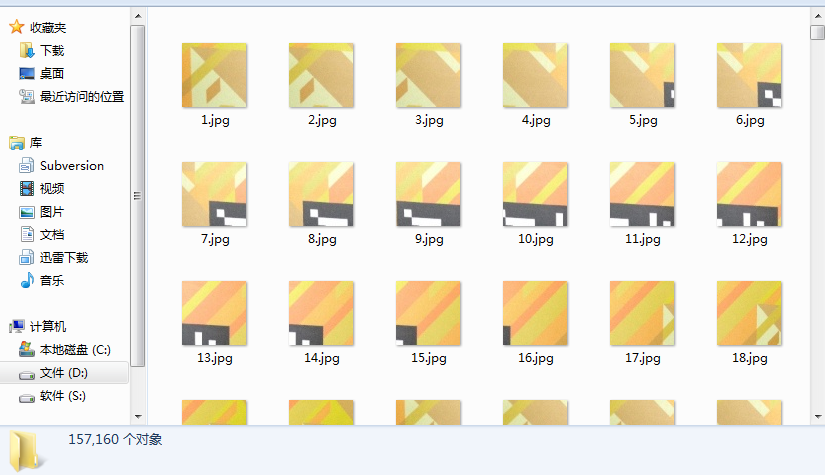利用hog+svm（梯度方向直方图和支持向量机）实现物体检测hog的话，相当于用一个滑动的窗口，在图像上从左到右，从上到下的滑动，每滑动到一个位置，计算其hog信息，重点要明白，这个hog信息，其实就是一个1*n的矩阵，说白了就是一个矢量，这个矢量就代表了这个窗口内的信息。

1.首先需要确定hog检测窗口的大小，这里以64*64像素大小的方框作为检测窗口。

1.为了让svm得到充足的训练，首先要准备大量的正负样本。这里我收集了各个姿态下的公仔的图片，共3000多张，这些样本都是正样本，且都为64*64像素大小。如图：2.同样的，还需要负样本，在没有公仔的场地内随意的拍照吧，大小也需要时64*64才行，共收集了15万张负样本图片，负样本图片里面都是不含有公仔的。（其实这里我偷了个懒，我用高分辨率的相机拍了很多照片，然后在这些照片中去截取64*64大小的像素块作为负样本，这样，一张普通的图片可以截取出上百张负样本图片，对于收集数据来说比较省事），如图：3.然后，将正负样本送入svm中进行训练，得到一个可以检测正负样本的结果。（看着数据量不少，几十万张图片，其实运算速度还好，我用个普通的电脑，这个训练的过程也就用了大概十分钟吧。其实，这只是个例子，真正要训练的话，这些数量的训练数据还是不够的）

4.然后就需要对图片中的物体进行检测了。以64*64的滑动窗口在图像上滑动，每滑动到一个位置，计算其hog特征，然后给svm产生的结果进行比较。如果方框里有我们要找的物体，那么理论上可以将这个hog特征归类为正样本的类别，这就完成了物体的检测。

5.由于滑动窗口移动的步长非常短，所以一个物体很有可能被检测出很多次，这些个检测出的框会密密麻麻的框住同一个物体。所以，后期还需要把这些同一个物体上的不同的框合成为一个。主要是根据框之间的距离和大小来进行方框的合并。最后合并完之后，就像下图一样：//#include<iostream>
//using namespace std;
//int main()
//{
//	cout<<"Hello World"<<endl;
//	return 0
//}
//#include "cui_hog_slow.h"
//#include"cui_hog.h"
#include<ctime>
#include <iostream>
#include <fstream>
#include <opencv2/core/core.hpp>
#include <opencv2/highgui/highgui.hpp>
#include <opencv2/imgproc/imgproc.hpp>
#include <opencv2/objdetect/objdetect.hpp>
#include <opencv2/ml/ml.hpp>

using namespace std;
using namespace cv;

#define squ 64       //方框的边长
#define PosSamNO 3513   //正样本个数
#define NegSamNO 157160    //负样本个数

#define TRAIN true    //是否进行训练,true表示重新训练，false表示读取xml文件中的SVM模型
#define CENTRAL_CROP true   //true:训练时，对96*160的INRIA正样本图片剪裁出中间的64*128大小人体

//HardExample：负样本个数。如果HardExampleNO大于0，表示处理完初始负样本集后，继续处理HardExample负样本集。
//不使用HardExample时必须设置为0，因为特征向量矩阵和特征类别矩阵的维数初始化时用到这个值
#define HardExampleNO 0

//继承自CvSVM的类，因为生成setSVMDetector()中用到的检测子参数时，需要用到训练好的SVM的decision_func参数，
//但通过查看CvSVM源码可知decision_func参数是protected类型变量，无法直接访问到，只能继承之后通过函数访问
class MySVM : public CvSVM
{
public:
//获得SVM的决策函数中的alpha数组
double * get_alpha_vector()
{
return this->decision_func->alpha;
}

//获得SVM的决策函数中的rho参数,即偏移量
float get_rho()
{
return this->decision_func->rho;
}
};

int main()
{

// //检测窗口(96,96),块尺寸(16,16),块步长(8,8),cell尺寸(8,8),直方图bin个数9
// HOGDescriptor hog(Size(squ,squ),Size(16,16),Size(8,8),Size(8,8),9);//HOG检测器，用来计算HOG描述子的
// int DescriptorDim;//HOG描述子的维数，由图片大小、检测窗口大小、块大小、细胞单元中直方图bin个数决定
// MySVM svm;//SVM分类器

// //若TRAIN为true，重新训练分类器
// if(TRAIN)
// {
//   //string ImgName;//图片名(绝对路径)
//   ifstream finPos("INRIAPerson96X160PosList.txt");//正样本图片的文件名列表
//   //ifstream finPos("PersonFromVOC2012List.txt");//正样本图片的文件名列表
//   ifstream finNeg("NoPersonFromINRIAList.txt");//负样本图片的文件名列表

//   Mat sampleFeatureMat;//所有训练样本的特征向量组成的矩阵，行数等于所有样本的个数，列数等于HOG描述子维数
//   Mat sampleLabelMat;//训练样本的类别向量，行数等于所有样本的个数，列数等于1；1表示有人，-1表示无人

//   //依次读取正样本图片，生成HOG描述子
//   //for(int num=1; num<(PosSamNO+1) && getline(finPos,ImgName); num++)
//for(int num=0; num<PosSamNO; num++)
//   {
//  string ImgName;//图片名(绝对路径)
//  stringstream stream;
//  stream<<(num+1);
//  ImgName=stream.str();
//     cout<<"处理："<<ImgName<<endl;
//     //ImgName = "D:\\DataSet\\PersonFromVOC2012\\" + ImgName;//加上正样本的路径名
//  ImgName = "D:\\file\\ylab_copy\\photo\\true_64\\" + ImgName+".jpg";
//  cout<<ImgName<<endl;
//     //ImgName = "D:\\DataSet\\INRIAPerson\\INRIAPerson\\96X160H96\\Train\\pos\\" + ImgName;//加上正样本的路径名
//     //if(CENTRAL_CROP)
//      //src = src(Rect(16,16,64,128));//将96*160的INRIA正样本图片剪裁为64*128，即剪去上下左右各16个像素
//     //resize(src,src,Size(64,128));

//     vector<float> descriptors;//HOG描述子向量
//     hog.compute(src,descriptors,Size(8,8));//计算HOG描述子，检测窗口移动步长(8,8)
//     //cout<<"描述子维数："<<descriptors.size()<<endl;

//     //处理第一个样本时初始化特征向量矩阵和类别矩阵，因为只有知道了特征向量的维数才能初始化特征向量矩阵
//     if( 0 == num )
//     {
//       DescriptorDim = descriptors.size();//HOG描述子的维数
//       //初始化所有训练样本的特征向量组成的矩阵，行数等于所有样本的个数，列数等于HOG描述子维数sampleFeatureMat
//       sampleFeatureMat = Mat::zeros(PosSamNO+NegSamNO+HardExampleNO, DescriptorDim, CV_32FC1);
//       //初始化训练样本的类别向量，行数等于所有样本的个数，列数等于1；1表示有人，0表示无人
//       sampleLabelMat = Mat::zeros(PosSamNO+NegSamNO+HardExampleNO, 1, CV_32FC1);
//     }

//     //将计算好的HOG描述子复制到样本特征矩阵sampleFeatureMat
//     for(int i=0; i<DescriptorDim; i++)
//       sampleFeatureMat.at<float>(num,i) = descriptors[i];//第num个样本的特征向量中的第i个元素
//     sampleLabelMat.at<float>(num,0) = 1;//正样本类别为1，有人
//   }

//   //依次读取负样本图片，生成HOG描述子
//   //for(int num=1; num<(NegSamNO+1) && getline(finNeg,ImgName); num++)
//for(int num=0; num<NegSamNO; num++)
//   {
//  string ImgName;//图片名(绝对路径)
//  stringstream stream;
//  stream<<(num+1);
//  ImgName=stream.str();
//     cout<<"处理："<<ImgName<<endl;
//  ImgName = "D:\\file\\ylab_copy\\photo\\false_64\\" + ImgName+".jpg";
//     //ImgName = "D:\\DataSet\\NoPersonFromINRIA\\" + ImgName;//加上负样本的路径名
//     //resize(src,img,Size(64,128));

//     vector<float> descriptors;//HOG描述子向量
//     hog.compute(src,descriptors,Size(8,8));//计算HOG描述子，检测窗口移动步长(8,8)
//     //cout<<"描述子维数："<<descriptors.size()<<endl;

//     //将计算好的HOG描述子复制到样本特征矩阵sampleFeatureMat
//     for(int i=0; i<DescriptorDim; i++)
//       sampleFeatureMat.at<float>(num+PosSamNO,i) = descriptors[i];//第PosSamNO+num个样本的特征向量中的第i个元素
//     sampleLabelMat.at<float>(num+PosSamNO,0) = -1;//负样本类别为-1，无人
//   }

//   //处理HardExample负样本
//   //if(HardExampleNO > 0)
//   //{
//   //  ifstream finHardExample("HardExample_2400PosINRIA_12000NegList.txt");//HardExample负样本的文件名列表
//   //  //依次读取HardExample负样本图片，生成HOG描述子
//   //  for(int num=0; num<HardExampleNO && getline(finHardExample,ImgName); num++)
//   //  {
//   //    cout<<"处理："<<ImgName<<endl;
//   //    ImgName = "D:\\DataSet\\HardExample_2400PosINRIA_12000Neg\\" + ImgName;//加上HardExample负样本的路径名
//   //    Mat src = imread(ImgName);//读取图片
//   //    //resize(src,img,Size(64,128));

//   //    vector<float> descriptors;//HOG描述子向量
//   //    hog.compute(src,descriptors,Size(8,8));//计算HOG描述子，检测窗口移动步长(8,8)
//   //    //cout<<"描述子维数："<<descriptors.size()<<endl;

//   //    //将计算好的HOG描述子复制到样本特征矩阵sampleFeatureMat
//   //    for(int i=0; i<DescriptorDim; i++)
//   //      sampleFeatureMat.at<float>(num+PosSamNO+NegSamNO,i) = descriptors[i];//第PosSamNO+num个样本的特征向量中的第i个元素
//   //    sampleLabelMat.at<float>(num+PosSamNO+NegSamNO,0) = -1;//负样本类别为-1，无人
//   //  }
//   //}

//   ////输出样本的HOG特征向量矩阵到文件
//   //ofstream fout("SampleFeatureMat.txt");
//   //for(int i=0; i<PosSamNO+NegSamNO; i++)
//   //{
//   //	fout<<i<<endl;
//   //	for(int j=0; j<DescriptorDim; j++)
//   //		fout<<sampleFeatureMat.at<float>(i,j)<<"  ";
//   //	fout<<endl;
//   //}

//   //训练SVM分类器
//   //迭代终止条件，当迭代满1000次或误差小于FLT_EPSILON时停止迭代
//   CvTermCriteria criteria = cvTermCriteria(CV_TERMCRIT_ITER+CV_TERMCRIT_EPS, 1000, FLT_EPSILON);
//   //SVM参数：SVM类型为C_SVC；线性核函数；松弛因子C=0.01
//   CvSVMParams param(CvSVM::C_SVC, CvSVM::LINEAR, 0, 1, 0, 0.01, 0, 0, 0, criteria);
//   cout<<"开始训练SVM分类器"<<endl;
//   svm.train(sampleFeatureMat, sampleLabelMat, Mat(), Mat(), param);//训练分类器
//   cout<<"训练完成"<<endl;
//   svm.save("SVM_HOG.xml");//将训练好的SVM模型保存为xml文件

// }
// else //若TRAIN为false，从XML文件读取训练好的分类器
// {
// }

// //*************************************************************************************************
// // 线性SVM训练完成后得到的XML文件里面，有一个数组，叫做support vector，还有一个数组，叫做alpha,有一个浮点数，叫做rho;
// //将alpha矩阵同support vector相乘，注意，alpha*supportVector,将得到一个列向量。之后，再该列向量的最后添加一个元素rho。
// //如此，变得到了一个分类器，利用该分类器，直接替换opencv中行人检测默认的那个分类器（cv::HOGDescriptor::setSVMDetector()），
// //就可以利用你的训练样本训练出来的分类器进行行人检测了。
// //***************************************************************************************************/
// DescriptorDim = svm.get_var_count();//特征向量的维数，即HOG描述子的维数
// int supportVectorNum = svm.get_support_vector_count();//支持向量的个数
// cout<<"支持向量个数："<<supportVectorNum<<endl;

// Mat alphaMat = Mat::zeros(1, supportVectorNum, CV_32FC1);//alpha向量，长度等于支持向量个数
// Mat supportVectorMat = Mat::zeros(supportVectorNum, DescriptorDim, CV_32FC1);//支持向量矩阵
// Mat resultMat = Mat::zeros(1, DescriptorDim, CV_32FC1);//alpha向量乘以支持向量矩阵的结果

// //将支持向量的数据复制到supportVectorMat矩阵中
// for(int i=0; i<supportVectorNum; i++)
// {
//   const float * pSVData = svm.get_support_vector(i);//返回第i个支持向量的数据指针
//   for(int j=0; j<DescriptorDim; j++)
//   {
//     //cout<<pData[j]<<" ";
//     supportVectorMat.at<float>(i,j) = pSVData[j];
//   }
// }

// //将alpha向量的数据复制到alphaMat中
// double * pAlphaData = svm.get_alpha_vector();//返回SVM的决策函数中的alpha向量
// for(int i=0; i<supportVectorNum; i++)
// {
// }

// //计算-(alphaMat * supportVectorMat),结果放到resultMat中
// //gemm(alphaMat, supportVectorMat, -1, 0, 1, resultMat);//不知道为什么加负号？
// resultMat = -1 * alphaMat * supportVectorMat;

// //得到最终的setSVMDetector(const vector<float>& detector)参数中可用的检测子
// vector<float> myDetector;
// //将resultMat中的数据复制到数组myDetector中
// for(int i=0; i<DescriptorDim; i++)
// {
//   myDetector.push_back(resultMat.at<float>(0,i));
// }
// //最后添加偏移量rho，得到检测子
// myDetector.push_back(svm.get_rho());
// cout<<"检测子维数："<<myDetector.size()<<endl;
// //设置HOGDescriptor的检测子
// HOGDescriptor myHOG(Size(squ,squ),Size(16,16),Size(8,8),Size(8,8),9);
// myHOG.setSVMDetector(myDetector);
// //myHOG.setSVMDetector(HOGDescriptor::getDefaultPeopleDetector());

// //保存检测子参数到文件
// ofstream fout("HOGDetector.txt");
// for(int i=0; i<myDetector.size(); i++)
// {
//   fout<<myDetector[i]<<endl;
// }
// cout<<"训练完成！"<<endl;

//**************读入图片进行HOG行人检测******************/

vector<float> myDetector;
float f;
String ds;
HOGDescriptor myHOG(Size(squ,squ),Size(16,16),Size(8,8),Size(8,8),9);
ifstream finPos("HOGDetector_64.txt");
while(getline(finPos,ds))
{
//cout<<ds<<endl;
f=atof(ds.c_str());
myDetector.push_back(f);
//cout<<f<<endl;
}
myHOG.setSVMDetector(myDetector);

Mat image;
resize(src,image,Size(src.cols/2,src.rows/2));

vector<Rect> found, found_filtered;//矩形框数组
clock_t t1,t2,t3;//用来统计程序的运行时间
t1=clock();
myHOG.detectMultiScale(image, found, 0, Size(8,8), Size(32,32), 1.07, 2);
//myHOG.detectMultiScale(image, found, 0, Size(8,8), Size(32,32), 1.05, 2);//对图片进行多尺度行人检测
cout<<"找到的矩形框个数："<<found.size()<<endl;
t2=clock();
//找出所有没有嵌套的矩形框r,并放入found_filtered中,如果有嵌套的话,则取外面最大的那个矩形框放入found_filtered中
for(int i=0; i < found.size(); i++)
{
Rect r = found[i];
int j=0;
for(; j < found.size(); j++)
if(j != i && (r & found[j]) == r)  //按位与操作
break;
if( j == found.size())
found_filtered.push_back(r);
}

for(int i=0; i<found.size(); i++)
{
Rect r = found[i];
//这里是对矩形框的大小和位置进行简单的调整，不要也罢
/* r.x += cvRound(r.width*0.1);
r.width = cvRound(r.width*0.8);
r.y += cvRound(r.height*0.07);
r.height = cvRound(r.height*0.8);*/
rectangle(image, r.tl(), r.br(), Scalar(0,255,0), 3);
}

cout << t2-t1   << "/" << CLOCKS_PER_SEC  << " (s) "<< endl;
cout << t3-t2   << "/" << CLOCKS_PER_SEC  << " (s) "<< endl;

//imwrite("ImgProcessed.jpg",src);

imshow("image",image);
//imwrite("D:\\file\\ylab_copy\\photo\\result\\64\\1.jpg",image);
waitKey(0);//

}